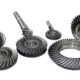###### Difference between Bevel gear and worm Gear
June 10, 2018June 25, 2019

## Gear TerminologyDiametral pitch (Pd): The number of teeth of a gear unit pitch diameter. The diametral pitch is, by definition, the number of teeth divided by the pitch diameter. That is,

Where

Pd = diametral pitch

N = number of teeth

D = pitch diameter

Pd      =        N/D

Module (m): Pitch diameter divided by number of teeth. The pitch diameter is usually specified in inches or millimetres; in the former case the module is the inverse of diametrical pitch.

m = D/N

Velocity ratio:-

d  = Diameter of the wheel

N =Speed of the wheel

w  = Angular speed